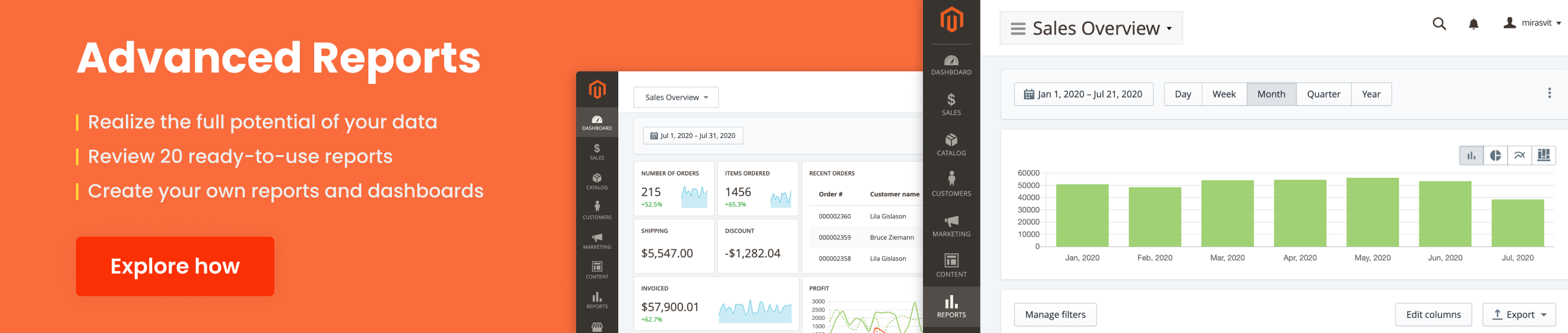# What does mean Gross Margin columnGross Margin is a special metric, which shows, how much in percentage company gains from each sales currency unit. It is calculated as Total Income minus Cost of Goods Sold, divided to Total Income.

Advanced Reports considers as Cost of Goods Sold value of Cost attribute, which you can see at Advanced Pricing section of product record.

Gross Margin, therefore, will be calculated as (Invoiced Subtotal - Base Cost) / Invoiced Subtotal.

Consider an order with a two products with Cost \$29. Product´s Special Price (i. e. actually paid by customer) is \$32, then Invoiced Subtotal would be \$32 * 2 = \$64. Base Cost would be \$29 * 2 = \$58. Then we have (\$64 - \$58) / \$64 = 0,938.

In percentage it would be 9,35% of Margin. This means, that company gained profit of 9,35% from each dollar, paid by customer in current order.

Gross Margin is calculated similarly. Extension calculates Margins for each order, and them sums them and displays average percent value for a period.

## Did you know…

… that you can define and even create your own metrics for your Reports. Our Advanced Reports come with a very flexible Report Builder component, that allows you to create reports for any activity on your store.

Moreover, using Config Builder you can even add your own columns with complex calculation, and even make your own definition of Gross Margin metric.